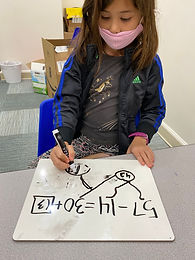Ms. Brianne

Target 1​

Lesson Type:

Continuation

Number Operation

:

Computation

Solve multi-step addition and subtraction problems within 50.

1:

Solve problems that have more than one operation, addition and subtraction.

2:

Understand when solving a problem that has both addition and subtraction operations that solving starts on the left and continues to the right.

2nd

Vocabulary:

Activities:

Students

• solved multi-step problems with both addition and subtraction
• used counting strategies, drawings, or fingers to help them as they moved from left to right in solving the equationHome Exploration

Challenge Problem:

There are 35 people on a train. At the first stop 12 more people got on the train and 7 people got off the train. How many people are on the train?

Guiding Questions:Absent Students:

Target 2

:

1:

Define an equal sign as a symbol which shows that the quantity on both sides are the same.

2:

Understand that an equal sign can link an equation to its solution or that it can link two equations together.

2nd

Vocabulary:

Activities:

Students

• were given equivalent equations with one term missing
• used counting strategies, fingers, drawings, and number lines to help them find the missing quantity
• created their own equivalent equations involving addition and subtractionHome Exploration

Guiding Questions:Target 3

:

1:

Match a given object to its silhouette (contour).

2:

Use observation skills to determine missing components.

1st

Vocabulary:

Activities:

Students

• matched each animal to its silhouette/shadow
• played a memory game with their animal and silhouette/shadow cards
• analyzed two pictures to find the differences between themHome Exploration